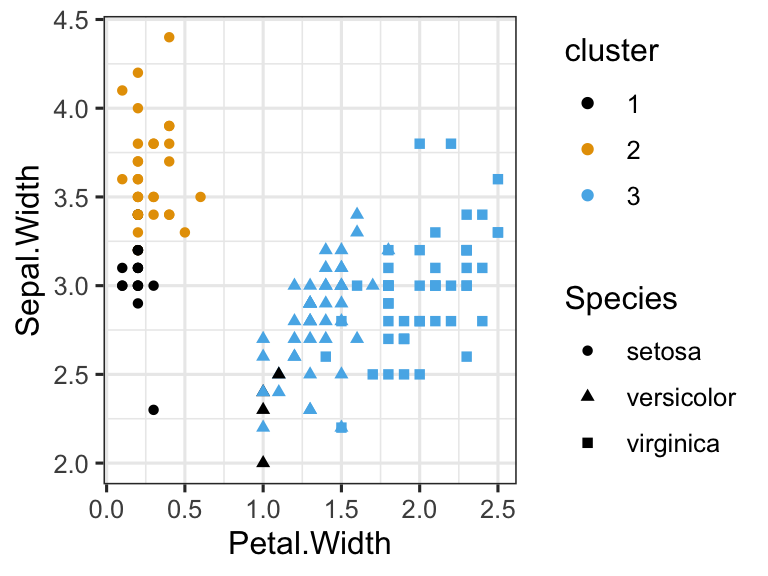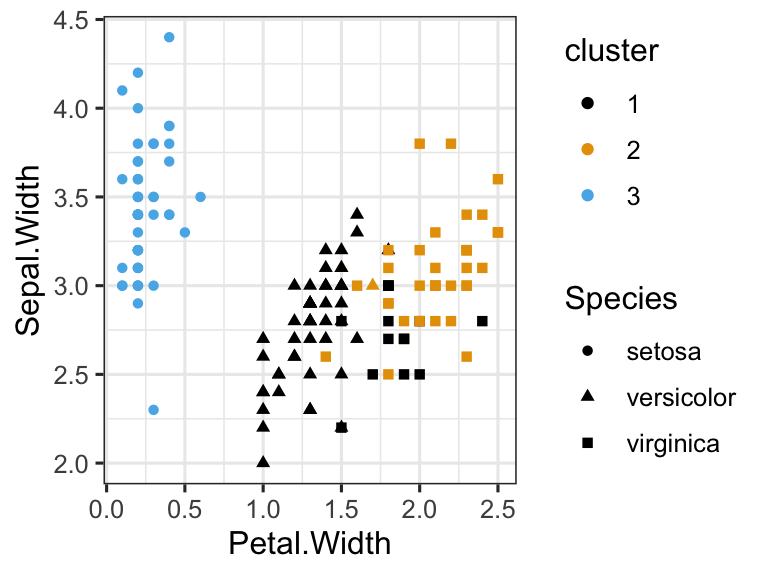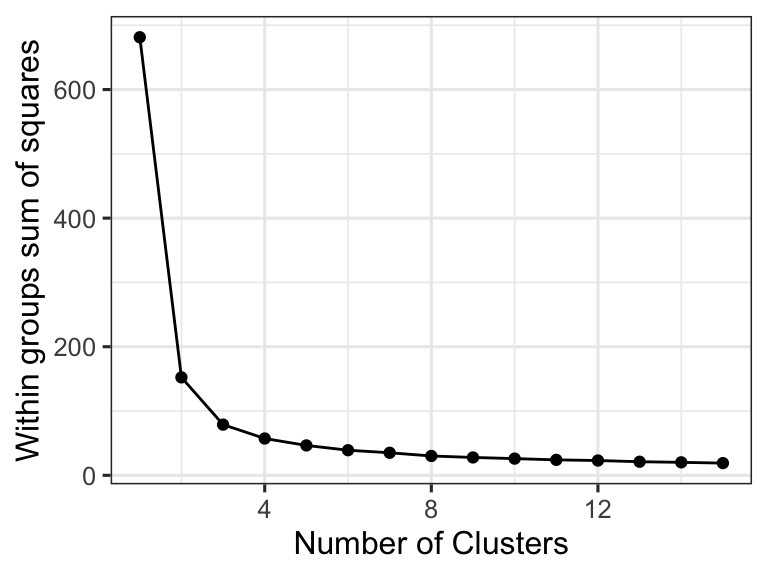## In-class worksheet 10

Feb 21, 2019

In this worksheet, we will use the libraries tidyverse and ggthemes:

``````library(tidyverse)
theme_set(theme_bw(base_size=12)) # set default ggplot2 theme
library(ggthemes)``````

# 1. k-means clustering of the iris data set

We can do k-means clustering in R using the function `kmeans()`. The primary argument to this function is `centers`, which tells the function how many cluster centers we want to use:

``````iris %>%
select(-Species) %>%    # remove Species column
kmeans(centers=3) ->    # do k-means clustering with 3 centers
km                      # store result as `km`

# now display the results from the analysis
km``````
``````## K-means clustering with 3 clusters of sizes 21, 33, 96
##
## Cluster means:
##   Sepal.Length Sepal.Width Petal.Length Petal.Width
## 1     4.738095    2.904762     1.790476   0.3523810
## 2     5.175758    3.624242     1.472727   0.2727273
## 3     6.314583    2.895833     4.973958   1.7031250
##
## Clustering vector:
##    2 1 1 1 2 2 2 2 1 1 2 2 1 1 2 2 2 2 2 2 2 2 2 2 1 1 2 2 2 1 1 2 2 2 1
##   2 2 2 1 2 2 1 1 2 2 1 2 1 2 2 3 3 3 3 3 3 3 1 3 3 1 3 3 3 3 3 3 3 3 3
##   3 3 3 3 3 3 3 3 3 3 3 3 3 3 3 3 3 3 3 3 3 3 3 1 3 3 3 3 1 3 3 3 3 3 3
##  3 3 3 3 3 3 3 3 3 3 3 3 3 3 3 3 3 3 3 3 3 3 3 3 3 3 3 3 3 3 3 3 3 3 3
##  3 3 3 3 3 3 3 3 3 3
##
## Within cluster sum of squares by cluster:
##   17.669524   6.432121 118.651875
##  (between_SS / total_SS =  79.0 %)
##
## Available components:
##
##  "cluster"      "centers"      "totss"        "withinss"
##  "tot.withinss" "betweenss"    "size"         "iter"
##  "ifault"``````

The cluster means give us the locations of the centroids of the three clusters. We can access them directly via `km\$centers`:

``km\$centers``
``````##   Sepal.Length Sepal.Width Petal.Length Petal.Width
## 1     4.738095    2.904762     1.790476   0.3523810
## 2     5.175758    3.624242     1.472727   0.2727273
## 3     6.314583    2.895833     4.973958   1.7031250``````

The clustering vector tells us for each data point to which cluster it belongs. We can access this vector directly via `km\$cluster`:

``km\$cluster``
``````##    2 1 1 1 2 2 2 2 1 1 2 2 1 1 2 2 2 2 2 2 2 2 2 2 1 1 2 2 2 1 1 2 2 2 1
##   2 2 2 1 2 2 1 1 2 2 1 2 1 2 2 3 3 3 3 3 3 3 1 3 3 1 3 3 3 3 3 3 3 3 3
##   3 3 3 3 3 3 3 3 3 3 3 3 3 3 3 3 3 3 3 3 3 3 3 1 3 3 3 3 1 3 3 3 3 3 3
##  3 3 3 3 3 3 3 3 3 3 3 3 3 3 3 3 3 3 3 3 3 3 3 3 3 3 3 3 3 3 3 3 3 3 3
##  3 3 3 3 3 3 3 3 3 3``````

We can add this vector to the iris data frame and then plot, coloring by cluster and using different symbol shapes according to species, for comparison:

``````# we use `factor(km\$cluster)` to tell R that the cluster numbers represent distinct categories,
# not continuous values
iris_clustered <- data.frame(iris, cluster = factor(km\$cluster))
ggplot(
iris_clustered,
aes(x = Petal.Width, y = Sepal.Width, color = cluster, shape = Species)
) +
geom_point() +
scale_color_colorblind()``````Let’s do everything once more:

``````iris %>% select(-Species) %>% kmeans(centers = 3) -> km
iris_clustered <- data.frame(iris, cluster = factor(km\$cluster))
ggplot(
iris_clustered,
aes(x = Petal.Width, y = Sepal.Width, color = cluster, shape = Species)
) +
geom_point() +
scale_color_colorblind()``````You see that the results do not look the same. This happens because the k-means algorithm uses a random starting point. To make the results less random, we can set the parameter `nstart` to a value other than 1, e.g. `nstart=10`:

``````iris %>% select(-Species) %>% kmeans(centers = 3, nstart = 10) -> km
iris_clustered <- data.frame(iris, cluster = factor(km\$cluster))
ggplot(
iris_clustered,
aes(x = Petal.Width, y = Sepal.Width, color = cluster, shape = Species)
) +
geom_point() +
scale_color_colorblind()``````To determine the best number of clusters in a systematic way, we can plot the within-groups sum of squares against the number of clusters and look for a characteristic slowdown in the decline:

``````iris_numeric <- select(iris, -Species) # make a data table with only the numeric measurements from iris

# calculate the weighted sum squares (wss) for a single cluster
wss <- (nrow(iris_numeric)-1)*sum(apply(iris_numeric, 2, var))

# now calculate the weighted sum squares for all cluster numbers from 2 to 15
for (i in 2:15) {
wss[i] <- sum(
kmeans(
iris_numeric,
nstart = 10,
centers = i
)\$withinss
)
}

# turn wss data into a data frame for plotting
wss_data <- data.frame(centers = 1:15, wss)

# make plot
ggplot(wss_data, aes(x = centers, y = wss)) +
geom_point() +
geom_line() +
xlab("Number of Clusters") +
ylab("Within groups sum of squares")``````We see that around 3 clusters, the decline slows down a lot. We would then choose 3 or 4 clusters for this data set.

# 2. Clustering the biopsy data set

Take the `biopsy` data set, cluster it into 2, 3, or 4 clusters, and display the clusters in principal-component space. (In other words, plot PC2 vs PC1 and color by cluster. You can set the plotting symbol by outcome, to see how clustering compares with known outcomes.)

``````# load in the biopsy data set
``````##   clump_thickness uniform_cell_size uniform_cell_shape marg_adhesion
## 1               5                 1                  1             1
## 2               5                 4                  4             5
## 3               3                 1                  1             1
## 4               6                 8                  8             1
## 5               4                 1                  1             3
## 6               8                10                 10             8
##   epithelial_cell_size bare_nuclei bland_chromatin normal_nucleoli mitoses
## 1                    2           1               3               1       1
## 2                    7          10               3               2       1
## 3                    2           2               3               1       1
## 4                    3           4               3               7       1
## 5                    2           1               3               1       1
## 6                    7          10               9               7       1
##     outcome
## 1    benign
## 2    benign
## 3    benign
## 4    benign
## 5    benign
## 6 malignant``````
``````# do PCA on biopsy data set
biopsy %>%
select(-outcome) %>%
scale() %>%
prcomp() ->
pca``````

Hint: To create the plot, you will have to make a new data frame out of the pca coordinates (`pca\$x`) and the clustering results (`km\$cluster`).

``# your R code goes here``

# 3. If this was easy

For the `biopsy` dataset, plot the within-group sum of squares against the number of clusters, to determine the appropriate number of clusters.

``# your R code goes here``

Now, go back to the original clustering of the `iris` data set, with 3 clusters:

``````iris %>%
select(-Species) %>%
kmeans(centers = 3, nstart = 10) ->
km

iris_clustered <- data.frame(iris, cluster = factor(km\$cluster))

ggplot(
iris_clustered,
aes(x = Petal.Width, y = Sepal.Width, color = cluster, shape = Species)
) +
geom_point() +
scale_color_colorblind()``````